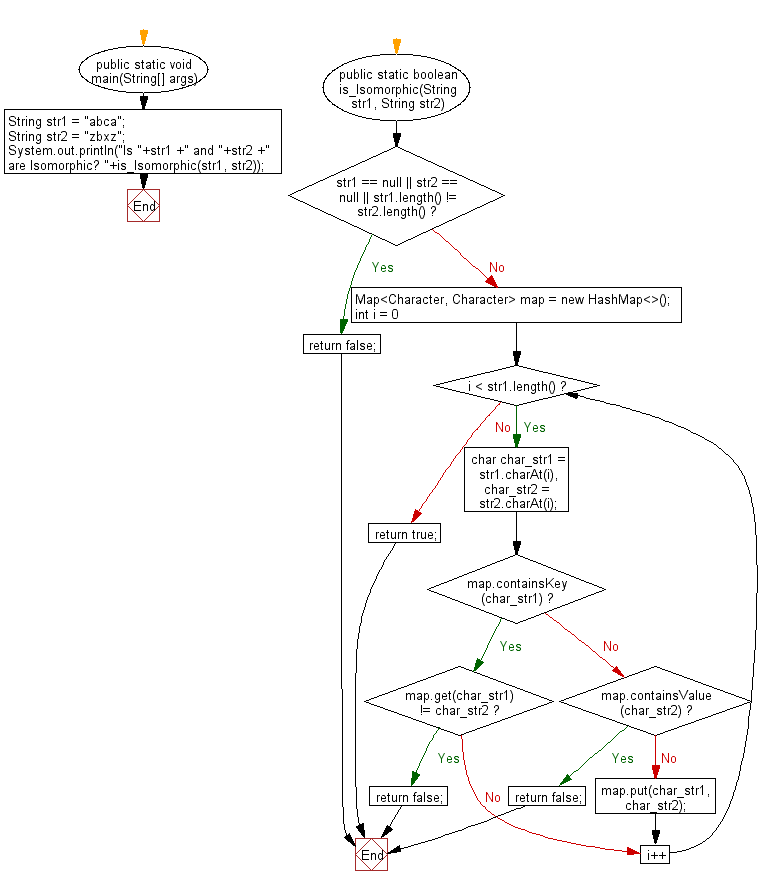﻿ Java programming exercises: Check if two specified strings are isomorphic or not - w3resource# Java Exercises: Check if two specified strings are isomorphic or not

## Java Basic: Exercise-185 with Solution

Write a Java program to check if two given strings are isomorphic or not.

Two strings are called isomorphic if the letters in one string can be remapped to get the second string. Remapping a letter means replacing all occurrences of it with another letter but the ordering of the letters remains unchanged. No two letters may map to the same letter, but a letter may map to itself.
Example-1: The words "abca" and "zbxz" are isomorphic because we can map 'a' to 'z', 'b' to 'b' and 'c' to 'x'.
Example-1: The words "bar" and "foo" are not isomorphic because we can map 'f' to 'b', 'o' to 'a' and 'o' to 'r'.

Sample Solution:

Java Code:

``````import java.util.*;
public class Solution {
public static void main(String[] args) {
String str1 = "abca";
String str2 = "zbxz";
System.out.println("Is "+str1 +" and "+str2 +" are Isomorphic? "+is_Isomorphic(str1, str2));
}

public static boolean is_Isomorphic(String str1, String str2) {
if (str1 == null || str2 == null || str1.length() != str2.length())
return false;
Map<Character, Character> map = new HashMap<>();

for (int i = 0; i < str1.length(); i++) {
char char_str1 = str1.charAt(i), char_str2 = str2.charAt(i);
if (map.containsKey(char_str1))
{
if (map.get(char_str1) != char_str2)
return false;
}
else
{
if (map.containsValue(char_str2))
return false;
map.put(char_str1, char_str2);
}
}

return true;
}
}
```
```

Sample Output:

```Is abca and zbxz are Isomorphic? true
```

Flowchart:Java Code Editor:

Company:

What is the difficulty level of this exercise?

﻿

## Java: Tips of the Day

Parsing dates:

```import java.io.*;
import java.util.*;
import java.text.*;

String s = "2001/09/23 14:39";

SimpleDateFormat formatter = new SimpleDateFormat ("yyyy/MM/dd H:mm");
Date d = formatter.parse(s, new ParsePosition(0));
```